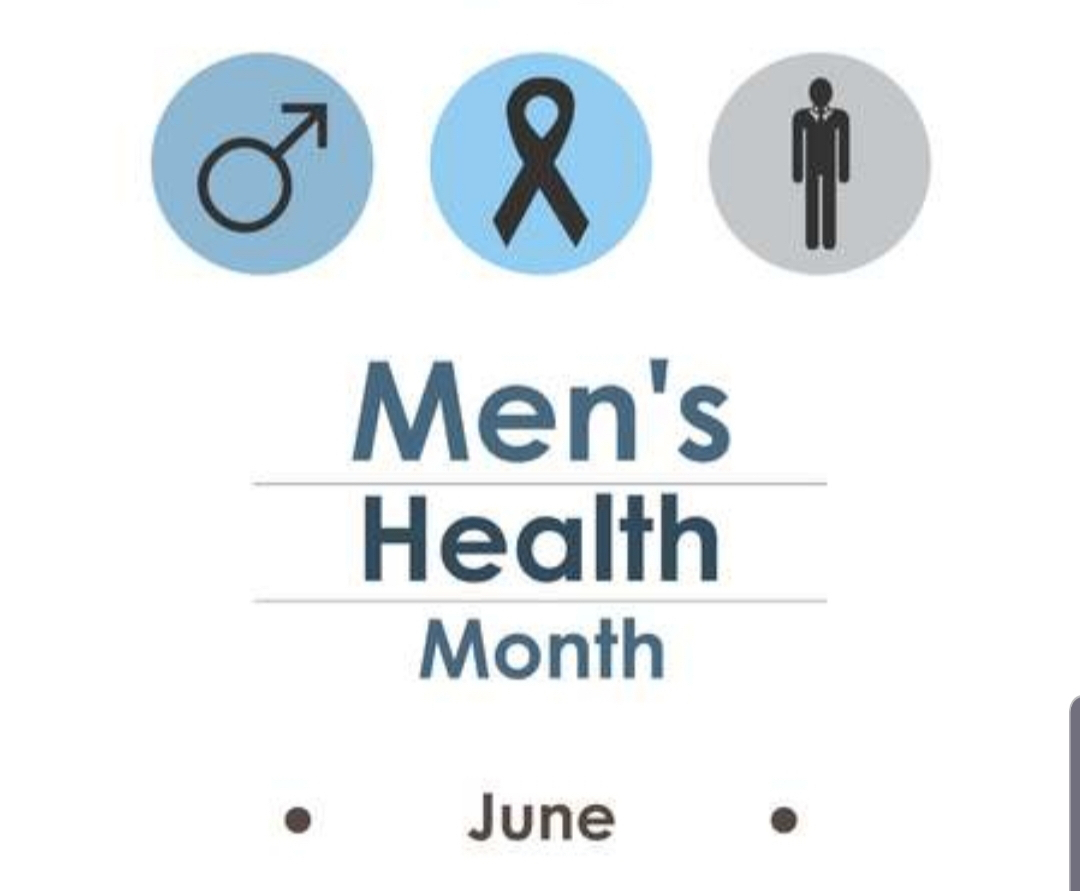## Resolution to Lifestyle

 /* styles */ No Matter The Day - It Will Be Music2YourFeet! -Denise Bryant, Founder
 table div table+table+table+table+table+table div table{width:100%;padding:0}table div table+table+table+table+table+table div table img{width:96.23%;padding:0;float:none}table div table+table+table+table+table+table div table td{width:100%;padding:0 1.88% 18px}/* styles *//* styles */
 table div table+table+table+table+table+table+table+table+table div table{width:100%;padding:0}table div table+table+table+table+table+table+table+table+table div table img{width:96.23%;padding:0;float:none}table div table+table+table+table+table+table+table+table+table div table td{width:100%;padding:0 1.88% 18px}/* styles */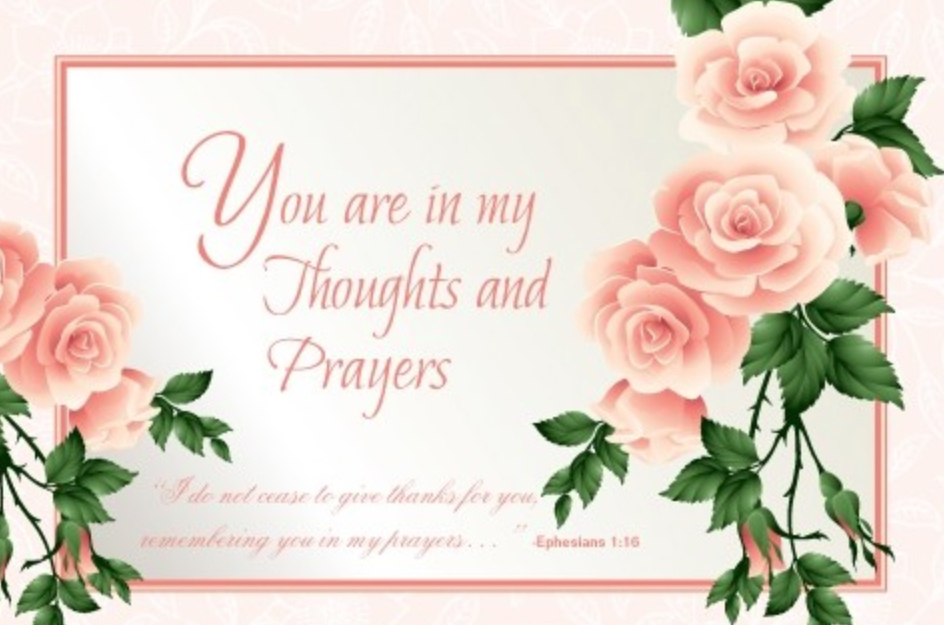/* styles */ My Condolences to George Floyd's family. God bless everyone who has lost moms, dads, sisters, brothers, family, friends, relatives, cousin's, co-workers, and line dance family due to Covid-19. Rest in Heaven, keep praying family.

## Denise's Corner

 table div table+table+table+table+table+table+table+table+table+table+table+table+table+table div table{width:100%;padding:0}table div table+table+table+table+table+table+table+table+table+table+table+table+table+table div table img{width:96.23%;padding:0;float:none}table div table+table+table+table+table+table+table+table+table+table+table+table+table+table div table td{width:100%;padding:0 1.88% 18px}/* styles */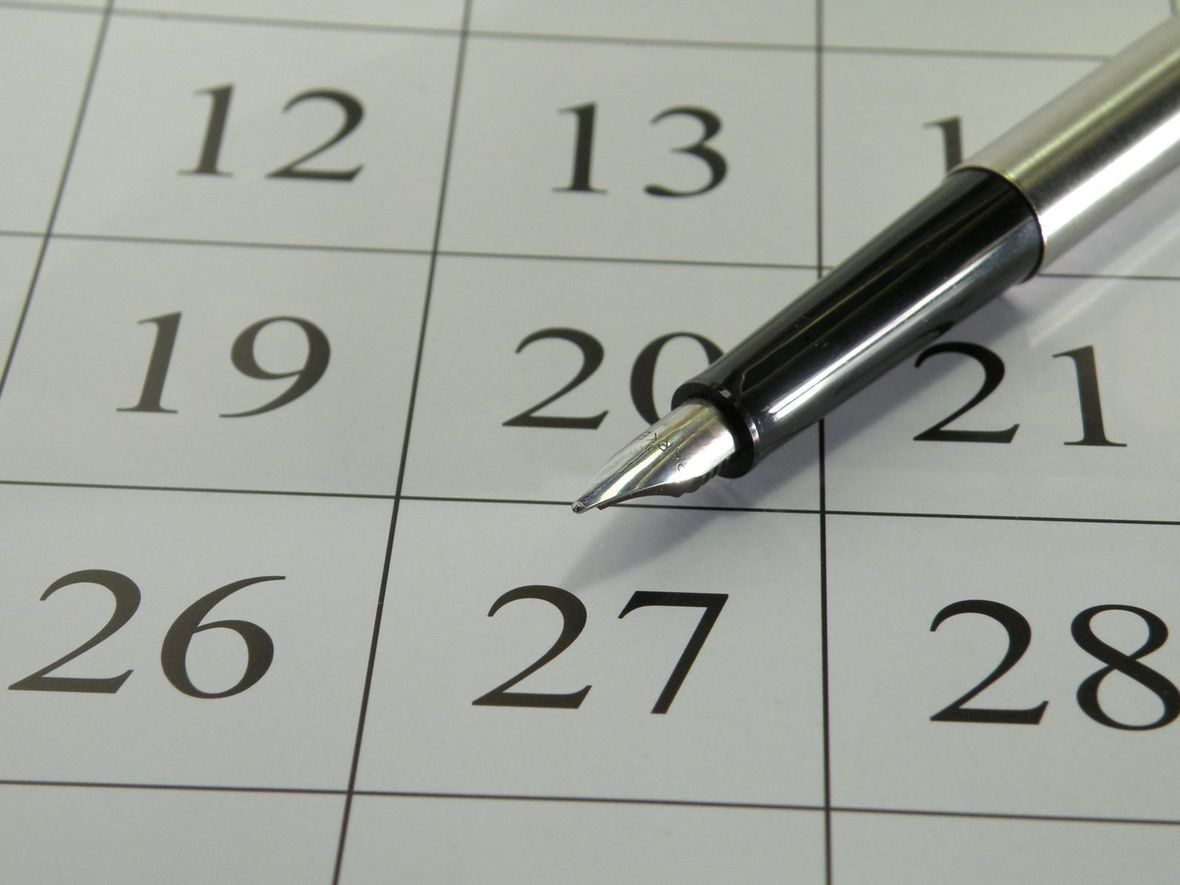# Music2yourfeet 7th Annual Holiday Party

 table div table+table+table+table+table+table+table+table+table+table+table+table+table+table+table+table div table{width:100%;padding:0}table div table+table+table+table+table+table+table+table+table+table+table+table+table+table+table+table div table img{width:96.23%;padding:0;float:none}table div table+table+table+table+table+table+table+table+table+table+table+table+table+table+table+table div table td{width:100%;padding:0 1.88% 18px}/* styles */## In the Spotlight...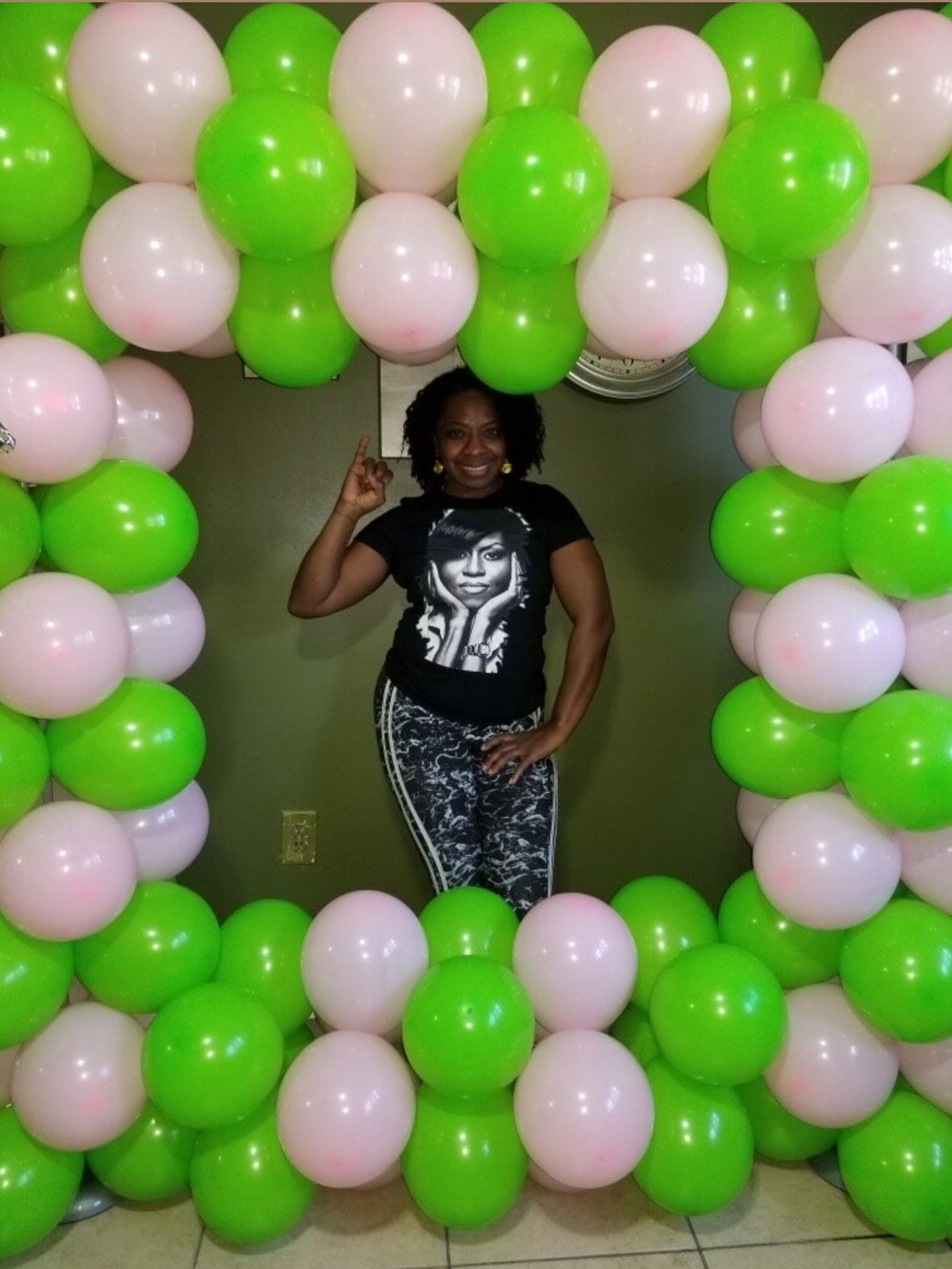# Celebrations By Suzie

What’s popping? My name is Glendora “Suzie” Franklin-Green and I have always had a knack for all things extravagant. My hobbies include line dancing, working out, and most of all: making your dreams come true!

Balloon art has quickly become my passion, but it is just the latest manifestation of my desire to create.

I have been an educator for over 13 years, but my creative side has always shone through. Throughout the years, I’ve decorated classrooms, planned multiple parties, and thrown events that are still being talked about to this day.

If you let Celebrations by Suzie decorate your event, you can rest assured it will be the best one yet. I won’t rest until the last balloon pops!

https://celebrationsbysuzie.com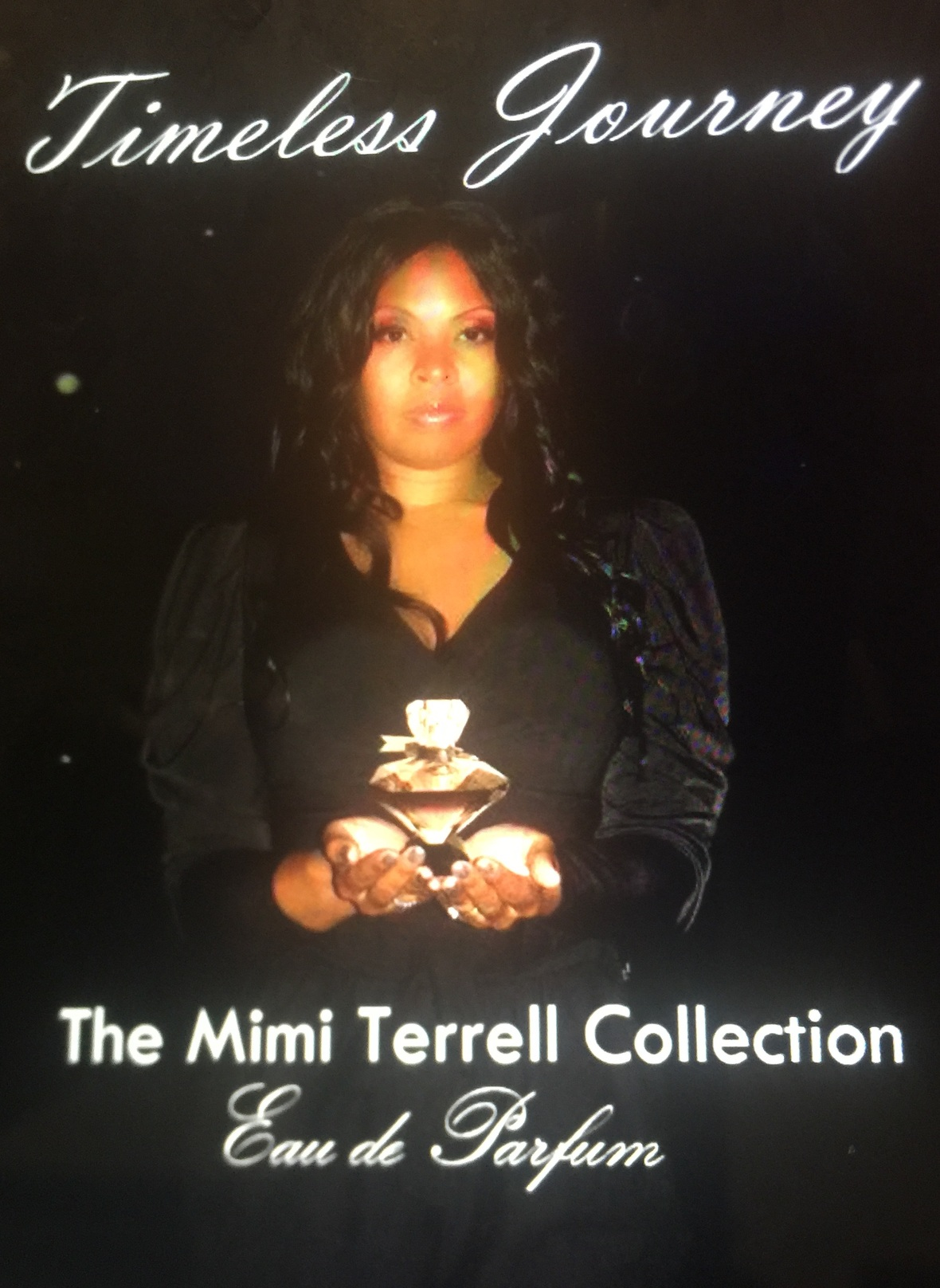# Tamika "Mimi" Starr

I have always dreamed of ecommerce plans for the future, my past times in general have been to test, own, and sell products that have personal feelings and experiences for me and my family. I sell an array of products, please connect with me to see my life experience spoken through my items.

If you purchase my products please feel free to shoot me a note and review of what you enjoyed!

Thanks again for supporting me and Team Tamika.

https://tamika-starr.my-online.store

 table div table+table+table+table+table+table+table+table+table+table+table+table+table+table+table+table+table+table+table+table div table{width:100%;padding:0}table div table+table+table+table+table+table+table+table+table+table+table+table+table+table+table+table+table+table+table+table div table img{width:96.23%;padding:0;float:none}table div table+table+table+table+table+table+table+table+table+table+table+table+table+table+table+table+table+table+table+table div table td{width:100%;padding:0 1.88% 18px}/* styles */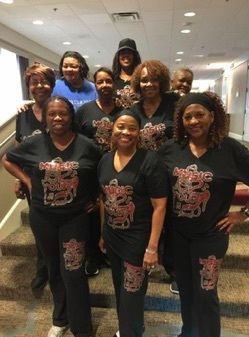Music2YourFeet Workout Suit (2 Piece)

\$40 for set, separate \$20 each,
come in black and gray only, stretch material...size from small to 2x pants and 3X shirt, may use Cash App \$seafo1 to pay, or cash, sorry no paypal and no checks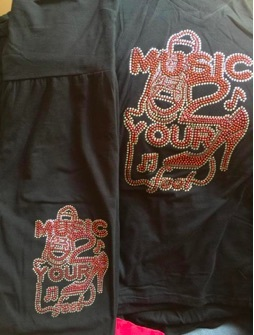Music2YourFeetWorkout Suit (pant and t-shirt)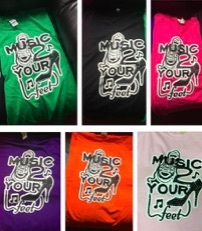Music2YourFeet T-Shirt

\$15 each, may use Cash App \$seafo1 to pay, or cash, sorry no paypal and no checks

Please indicate the T-shirt color when ordering.
Colors available:
Green, Black, Hot Pink, Purple, Orange and Light Pink,

 table div table+table+table+table+table+table+table+table+table+table+table+table+table+table+table+table+table+table+table+table+table+table+table+table+table div table{width:100%;padding:0}table div table+table+table+table+table+table+table+table+table+table+table+table+table+table+table+table+table+table+table+table+table+table+table+table+table div table img{width:96.23%;padding:0;float:none}table div table+table+table+table+table+table+table+table+table+table+table+table+table+table+table+table+table+table+table+table+table+table+table+table+table div table td{width:100%;padding:0 1.88% 18px}/* styles */## Special Birthday shout outs to my family and friends....

 /* styles */ A very special Happy Birthday to my nephew in Georgia, David Jackson, Enjoy your special day nephew, I love you!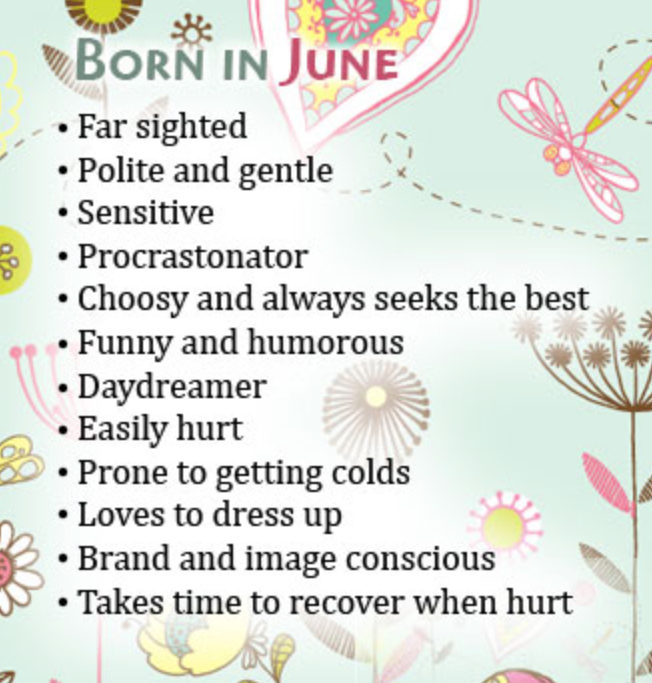table div table+table+table+table+table+table+table+table+table+table+table+table+table+table+table+table+table+table+table+table+table+table+table+table+table+table+table+table+table+table div table{width:100%;padding:0}table div table+table+table+table+table+table+table+table+table+table+table+table+table+table+table+table+table+table+table+table+table+table+table+table+table+table+table+table+table+table div table img{width:96.23%;padding:0;float:none}table div table+table+table+table+table+table+table+table+table+table+table+table+table+table+table+table+table+table+table+table+table+table+table+table+table+table+table+table+table+table div table td{width:100%;padding:0 1.88% 18px}/* styles */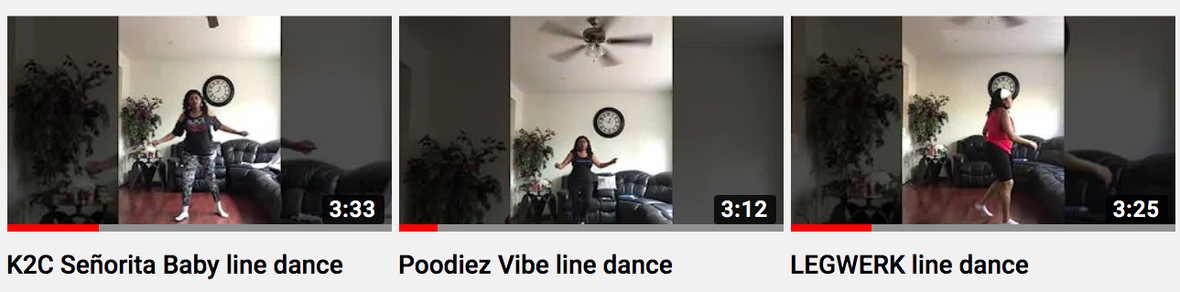/* styles */ View new line dance videos: http://www.music2yourfeet.com/videos.html
 /* styles */ Music2YourFeet visits many areas within the community, centers, parks and other events - spreading our love of line dancing. We capture every moment with video and photos. Please check out our photo gallery each month - you may find yourself in them - "Aroundtown"!
 table div table+table+table+table+table+table+table+table+table+table+table+table+table+table+table+table+table+table+table+table+table+table+table+table+table+table+table+table+table+table+table+table+table+table+table+table div table{width:100%;padding:0}table div table+table+table+table+table+table+table+table+table+table+table+table+table+table+table+table+table+table+table+table+table+table+table+table+table+table+table+table+table+table+table+table+table+table+table+table div table img{width:96.23%;padding:0;float:none}table div table+table+table+table+table+table+table+table+table+table+table+table+table+table+table+table+table+table+table+table+table+table+table+table+table+table+table+table+table+table+table+table+table+table+table+table div table td{width:100%;padding:0 1.88% 18px}/* styles *//* styles */ Special Thank you To front line employees who are helping to keep us safe, we love and appreciate you.table div table+table+table+table+table+table+table+table+table+table+table+table+table+table+table+table+table+table+table+table+table+table+table+table+table+table+table+table+table+table+table+table+table+table+table+table+table+table+table div table{width:100%;padding:0}table div table+table+table+table+table+table+table+table+table+table+table+table+table+table+table+table+table+table+table+table+table+table+table+table+table+table+table+table+table+table+table+table+table+table+table+table+table+table+table div table img{width:96.23%;padding:0;float:none}table div table+table+table+table+table+table+table+table+table+table+table+table+table+table+table+table+table+table+table+table+table+table+table+table+table+table+table+table+table+table+table+table+table+table+table+table+table+table+table div table td{width:100%;padding:0 1.88% 18px}/* styles */table.module-40{width:75.47%;padding:0}table div table+table+table+table+table+table+table+table+table+table+table+table+table+table+table+table+table+table+table+table+table+table+table+table+table+table+table+table+table+table+table+table+table+table+table+table+table+table+table+table+table div table{width:75.47%;float:none;margin-left:auto;margin-right:auto;padding:0}table div table+table+table+table+table+table+table+table+table+table+table+table+table+table+table+table+table+table+table+table+table+table+table+table+table+table+table+table+table+table+table+table+table+table+table+table+table+table+table+table+table div table a{border:0 none;text-decoration:none}table div table+table+table+table+table+table+table+table+table+table+table+table+table+table+table+table+table+table+table+table+table+table+table+table+table+table+table+table+table+table+table+table+table+table+table+table+table+table+table+table+table div table img{width:100%!important;border:0 none;text-decoration:none}table div table+table+table+table+table+table+table+table+table+table+table+table+table+table+table+table+table+table+table+table+table+table+table+table+table+table+table+table+table+table+table+table+table+table+table+table+table+table+table+table+table div table td{width:100%;padding:0}/* styles */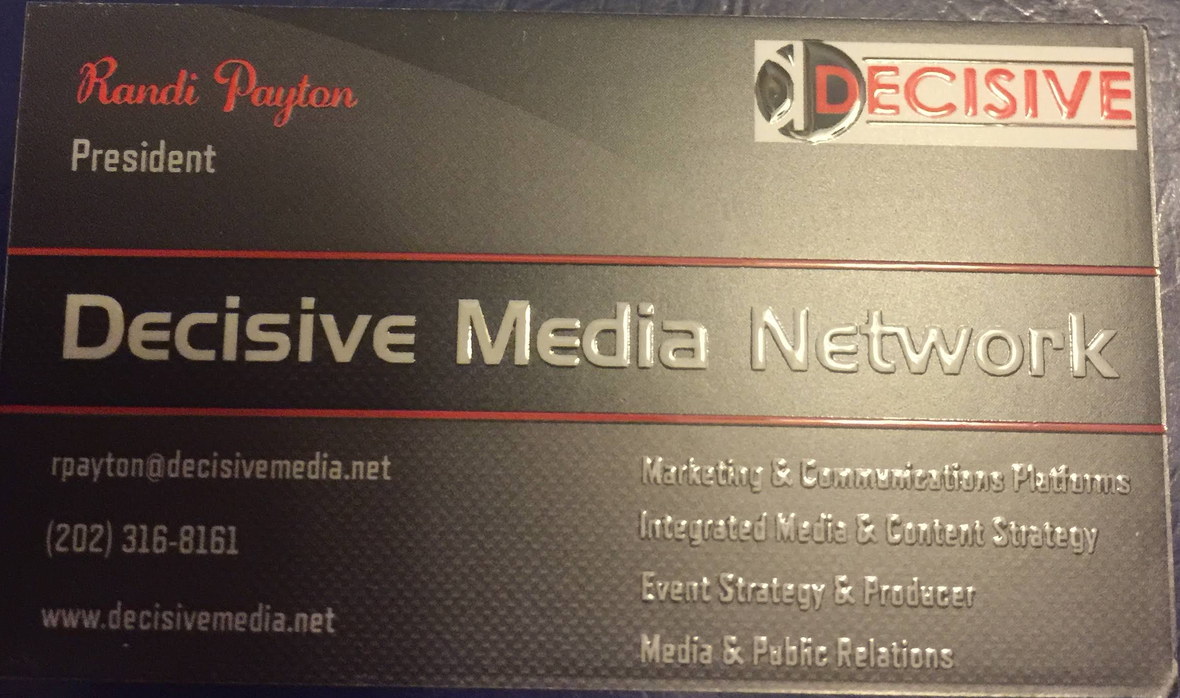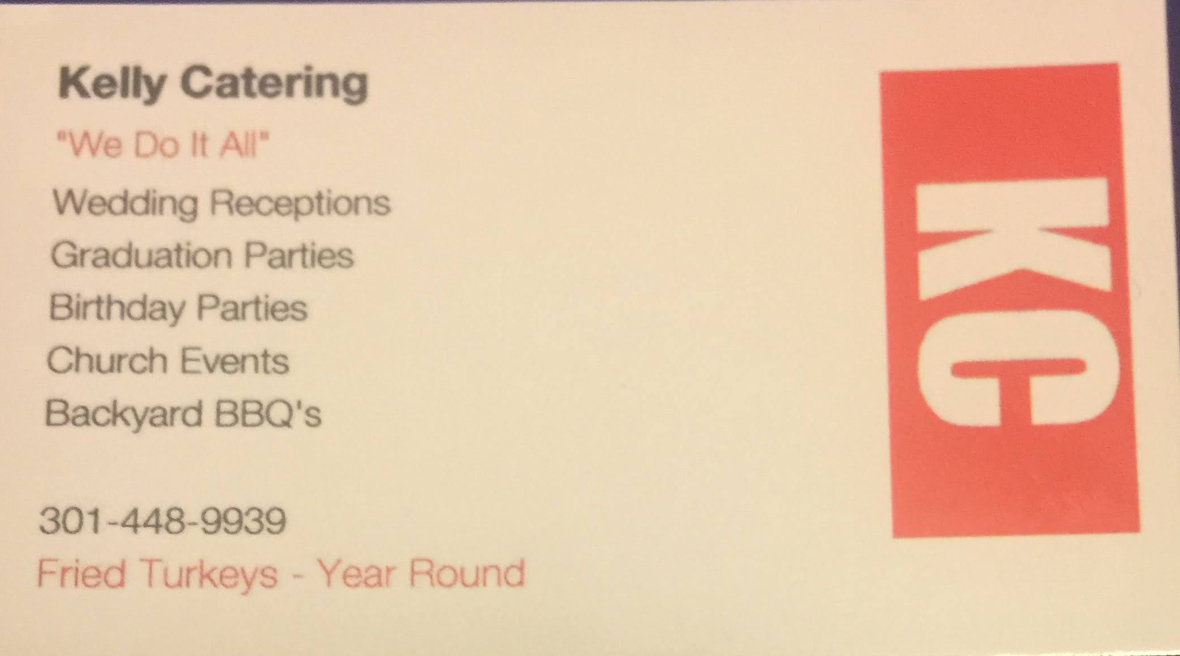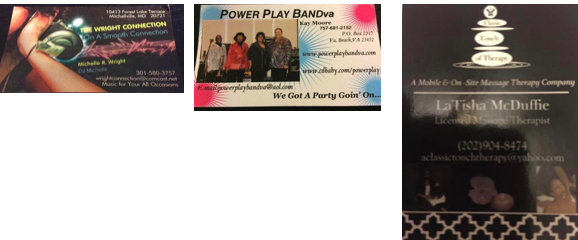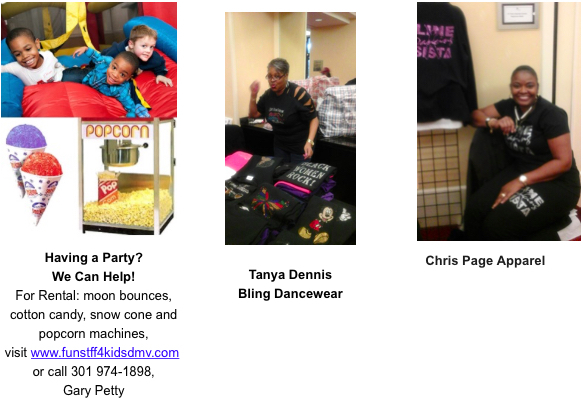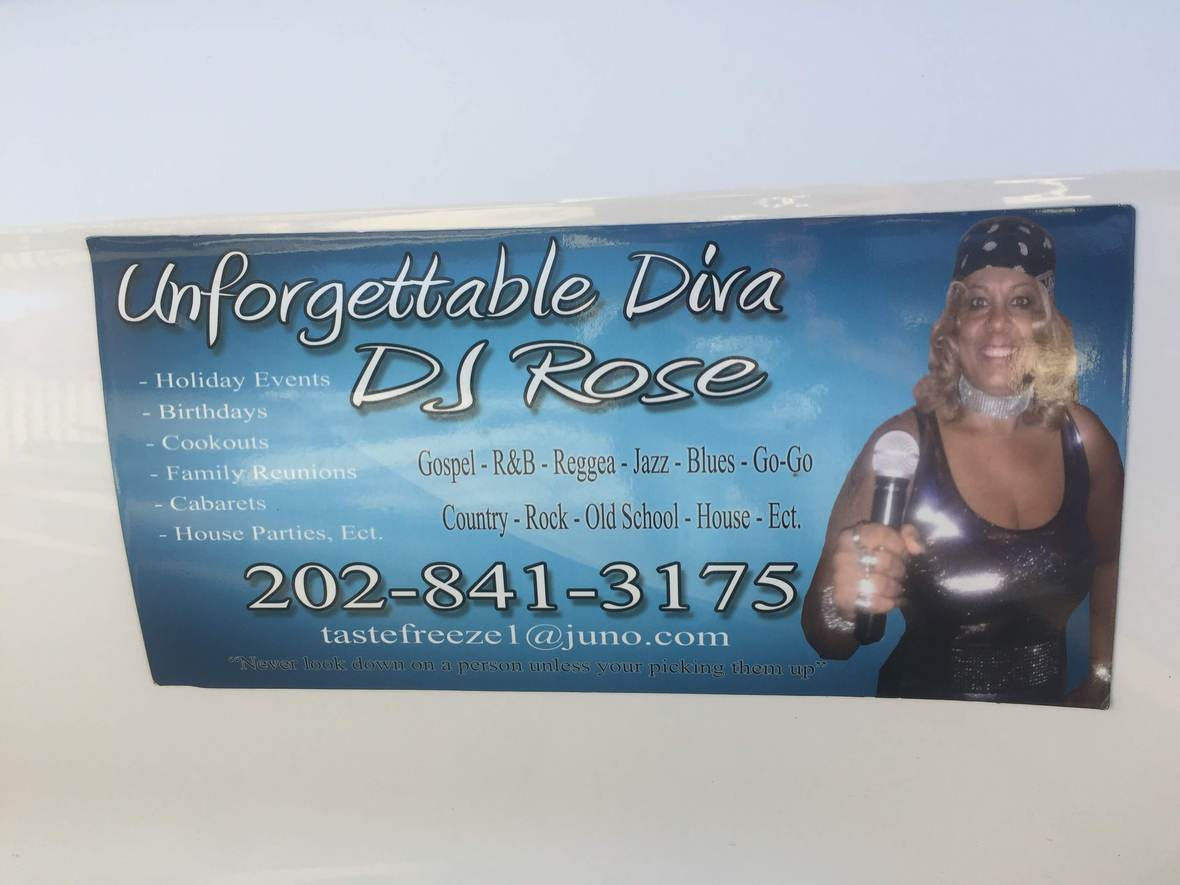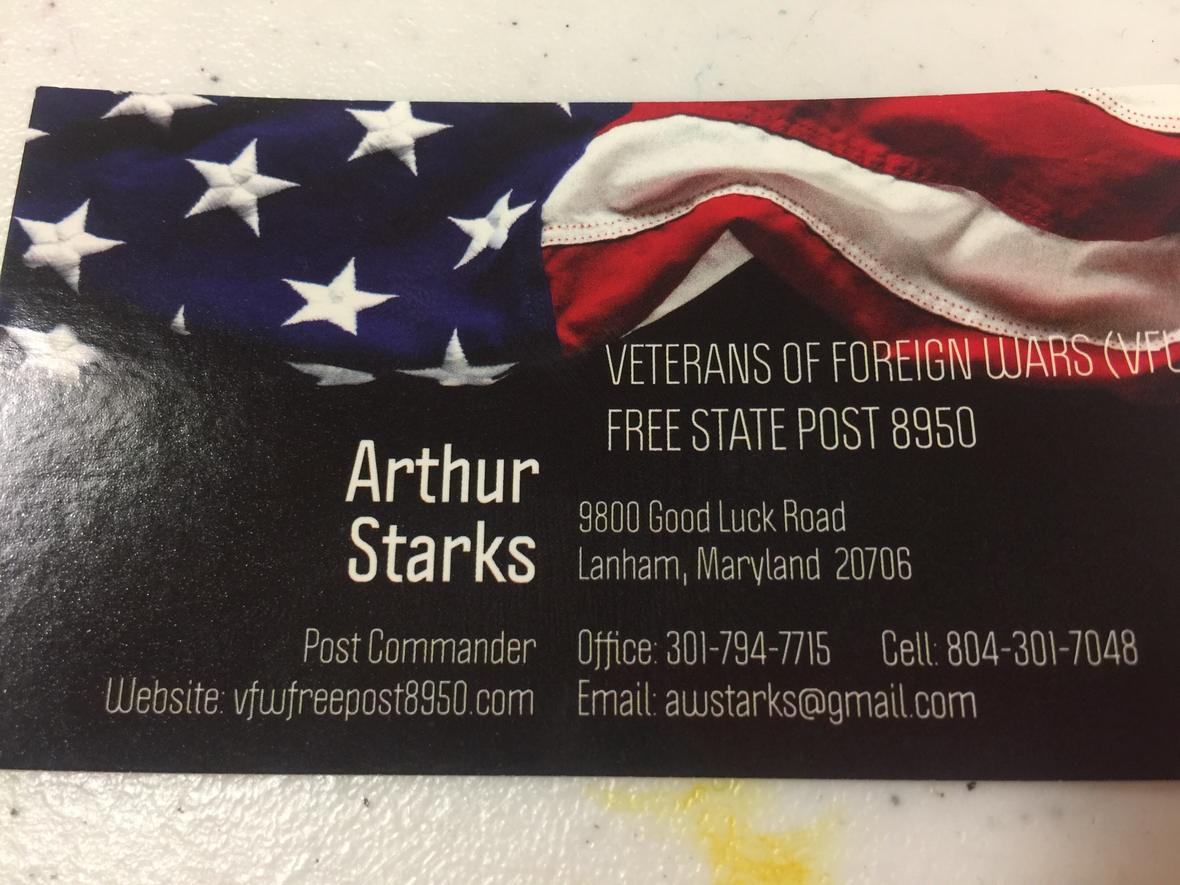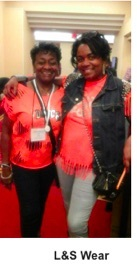# Thank you for your continued support!Classes and Social Events Cancelled Due to the Coronavirus Outbreak
As positive coronavirus cases have increased in Maryland and Gov. Larry Hogan (R) has declared a state of emergency, which has restricted public gatherings, businesses and government facilities, most public events have been canceled or postponed and public access to government services are increasingly restricted. With that all dance classes and social dance events are cancelled. Please check our website and Facebook page for updates.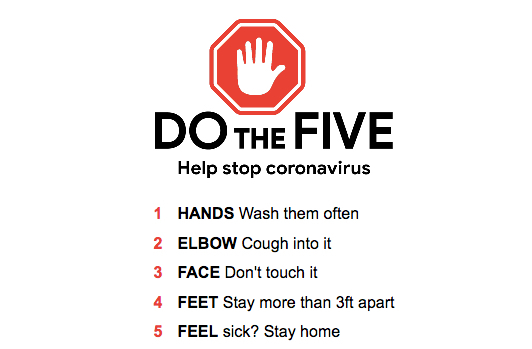table div table+table+table+table+table+table+table+table+table+table+table+table+table+table+table+table+table+table+table+table+table+table+table+table+table+table+table+table+table+table+table+table+table+table+table+table+table+table+table+table+table+table+table+table+table+table+table+table+table+table+table+table+table+table div table{width:100%;padding:0}table div table+table+table+table+table+table+table+table+table+table+table+table+table+table+table+table+table+table+table+table+table+table+table+table+table+table+table+table+table+table+table+table+table+table+table+table+table+table+table+table+table+table+table+table+table+table+table+table+table+table+table+table+table+table div table img{width:96.23%;padding:0;float:none}table div table+table+table+table+table+table+table+table+table+table+table+table+table+table+table+table+table+table+table+table+table+table+table+table+table+table+table+table+table+table+table+table+table+table+table+table+table+table+table+table+table+table+table+table+table+table+table+table+table+table+table+table+table+table div table td{width:100%;padding:0 1.88% 18px}/* styles */## Music2YourFeet Classes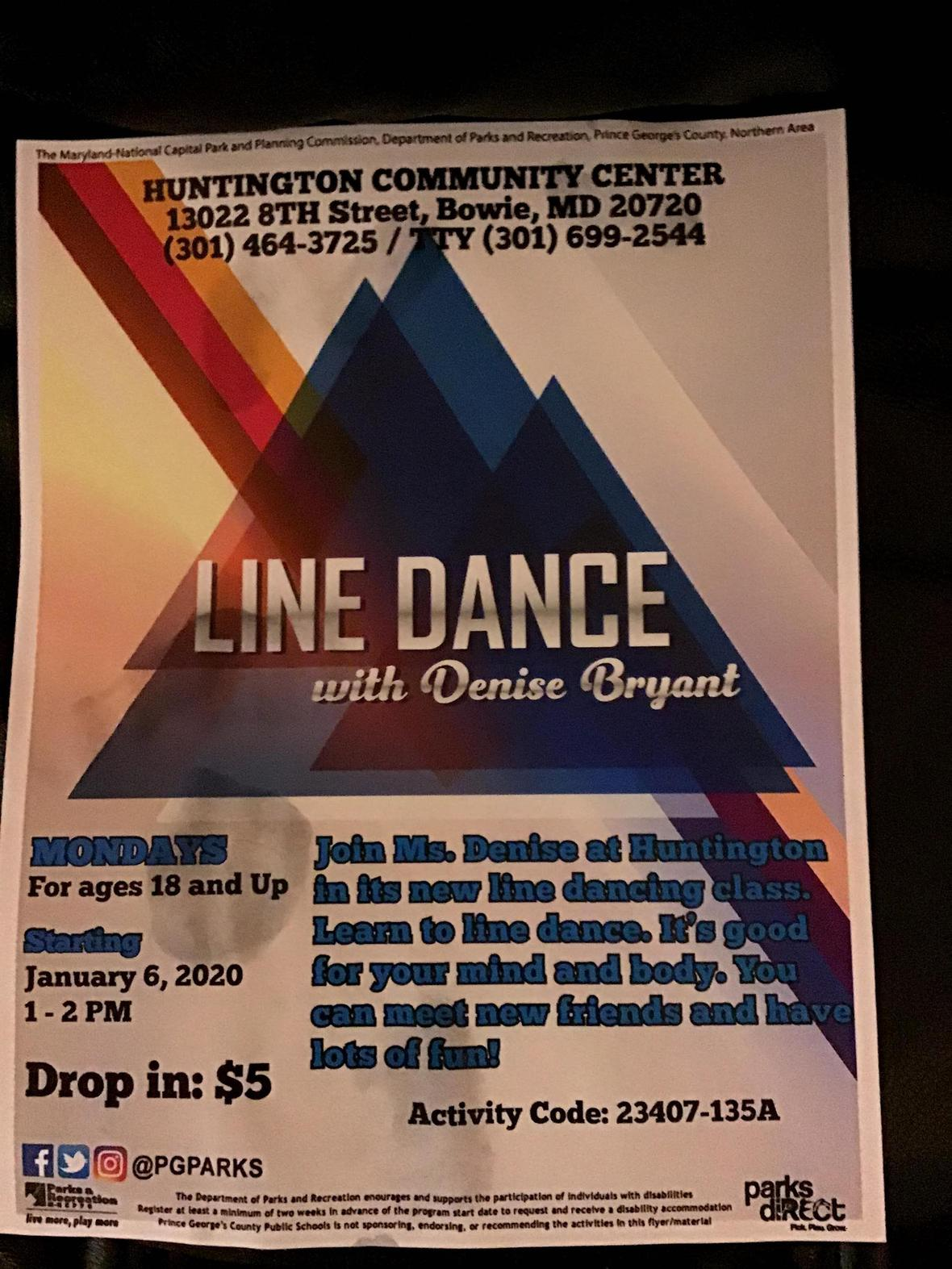Music2yourfeet line dance is at Huntington Community Center/Old Antique Bowie!

Beginners and Seniors, but all are welcome....come out for some fun and exercise, no experience necessary...every Monday at 1:00, see you there.

When: Mondays 1:00-2:00, \$5 drop in
Where: 13022 8th Street, Bowie, Md.

 /* styles */ Monday Lake Arbor Community Center 10100 Lake Arbor Way, Mitchellville, Md. 20721 10:00am-11:30am Calling all Beginners & Seniors, come out for some fun and exercise with Denise Monday Huntington Community Center 13022 8th Street Bowie, MD 1:00pm - 2:00pm \$5 drop in Tuesday Cedar Heights Community Center 1200 Glen Willow Drive Seat Pleasant, MD 9:00am-10:30am \$6, Intermediate Tuesday Glenn Dale Community Center 11901 Glenn Dale Blvd, Glenn Dale, MD 20769 11-12:30pm Beginner \$20 for residents and \$24 for Non-residents. \$5 drop in fee. Beginner Wednesday Good Luck Community Ctr. 6:30-8:00pm 8601 Good Luck Rd, Lanham, Md. 20706 Beginner Friday Ceder Heights Community Center 9am-10:30am 1200 Glen Willow Dr, Capitol Heights, MD 20743 Beginner Friday Senior Day Good Luck Community Center (on-going) 8601 Good Luck Rd Lanham, Md. 11:30am-1:30pm Seniors 60+ FREE, under 60 yrs. Old \$6 Music2YourFeet Private Lessons Available
 table div table+table+table+table+table+table+table+table+table+table+table+table+table+table+table+table+table+table+table+table+table+table+table+table+table+table+table+table+table+table+table+table+table+table+table+table+table+table+table+table+table+table+table+table+table+table+table+table+table+table+table+table+table+table+table+table+table+table+table div table{width:100%;padding:0}table div table+table+table+table+table+table+table+table+table+table+table+table+table+table+table+table+table+table+table+table+table+table+table+table+table+table+table+table+table+table+table+table+table+table+table+table+table+table+table+table+table+table+table+table+table+table+table+table+table+table+table+table+table+table+table+table+table+table+table div table img{width:96.23%;padding:0;float:none}table div table+table+table+table+table+table+table+table+table+table+table+table+table+table+table+table+table+table+table+table+table+table+table+table+table+table+table+table+table+table+table+table+table+table+table+table+table+table+table+table+table+table+table+table+table+table+table+table+table+table+table+table+table+table+table+table+table+table+table div table td{width:100%;padding:0 1.88% 18px}/* styles */## DMV Monthly Dance Socials

 /* styles */ First Sunday of Each Month Sunday Line Dance Social - at Cedar Heights Community Center, 1200 Glen Willow Drive, Seat Pleasant, Md. 3pm - 7pm \$6 for Res & Non-Res. First Friday Line Dance Socials with April Love Jones Southern Regional Technology and Recreation Complex, 6pm-9:30pm includes light refreshments, \$5 resident \$6 non resident First and Third Friday Delight featuring DJ Zone @ VFW Post 7916 (upstairs), 204 Mill Street Occoquan, Va 22125. Time: 7:30 PM to 11:30 PM. Cost: \$10. Open dancing, Cash Bar, Bring your own Food or purchase from the VFW. Follow DJ Zone on Facebook under Vincent Price and join my DJ Zone Status Group for other events and dates. First Saturday of Each Month - Toast it Up Productions - Shut Up & Dance, 1:00-5:00pm, Elk's Lodge, 7350 Temple Hills, Md. BYOF, \$7.00 Third Saturday of Each Month S.O.S. (Seniors On Step) line dance social; 6:00-10:00pm; Cost: Free; BYOFood & beverage (no alcohol permitted) Kingstowne Community Center for Active Adults, 6488 Landsdowne Centre Drive, Alexandria, VA 22315. Every Second Friday, \$5 6p-9p. Learn the latest line dances with Peytrienne! All are welcome, water provided, Soul Line & Hand dance Party, White Oak Community Center, 1700 April Lane Silver Spring, Md. 20904 Every Sunday Elks Lodge of Camp Springs 7350 Temple Hills Rd Temple Hills Md, Hand Dance, Bop, Line Dance & more 6p-10p Fourth Friday of the Month - Style & Rhythm, 4th Friday social ,\$5, Ridgley's Run Community Ctr. 8400 Mission Road, Jessup, Md.
 table div table+table+table+table+table+table+table+table+table+table+table+table+table+table+table+table+table+table+table+table+table+table+table+table+table+table+table+table+table+table+table+table+table+table+table+table+table+table+table+table+table+table+table+table+table+table+table+table+table+table+table+table+table+table+table+table+table+table+table+table+table+table div table{width:100%;padding:0}table div table+table+table+table+table+table+table+table+table+table+table+table+table+table+table+table+table+table+table+table+table+table+table+table+table+table+table+table+table+table+table+table+table+table+table+table+table+table+table+table+table+table+table+table+table+table+table+table+table+table+table+table+table+table+table+table+table+table+table+table+table+table div table img{width:96.23%;padding:0;float:none}table div table+table+table+table+table+table+table+table+table+table+table+table+table+table+table+table+table+table+table+table+table+table+table+table+table+table+table+table+table+table+table+table+table+table+table+table+table+table+table+table+table+table+table+table+table+table+table+table+table+table+table+table+table+table+table+table+table+table+table+table+table+table div table td{width:100%;padding:0 1.88% 18px}/* styles *//* styles */ Denise's "Music2YourFeet" Line Dancing provides you with the opportunity to exercise socialize and have fun - all in one setting. Break the monotony of a long day at work, (evening classes); get mid-day relief to an otherwise stressful day (lunch time classes); Relax after a long work week (weekend classes); or one-on-one personal service (private classes, no matter the day, it will be music2yourfeet Submit your events. Donations not required but appreciated.
 table div table+table+table+table+table+table+table+table+table+table+table+table+table+table+table+table+table+table+table+table+table+table+table+table+table+table+table+table+table+table+table+table+table+table+table+table+table+table+table+table+table+table+table+table+table+table+table+table+table+table+table+table+table+table+table+table+table+table+table+table+table+table+table+table+table div table{width:100%;padding:0}table div table+table+table+table+table+table+table+table+table+table+table+table+table+table+table+table+table+table+table+table+table+table+table+table+table+table+table+table+table+table+table+table+table+table+table+table+table+table+table+table+table+table+table+table+table+table+table+table+table+table+table+table+table+table+table+table+table+table+table+table+table+table+table+table+table div table img{width:96.23%;padding:0;float:none}table div table+table+table+table+table+table+table+table+table+table+table+table+table+table+table+table+table+table+table+table+table+table+table+table+table+table+table+table+table+table+table+table+table+table+table+table+table+table+table+table+table+table+table+table+table+table+table+table+table+table+table+table+table+table+table+table+table+table+table+table+table+table+table+table+table div table td{width:100%;padding:0 1.88% 18px}/* styles */## More Line Dance Classes

 /* styles */ Garland Christopher Tuesday only class 11:00a-12:00pm Palmer Park Community Center 7720 Barlowe Rd, Landover, MD 20785 (301) 773-5665
 table div table+table+table+table+table+table+table+table+table+table+table+table+table+table+table+table+table+table+table+table+table+table+table+table+table+table+table+table+table+table+table+table+table+table+table+table+table+table+table+table+table+table+table+table+table+table+table+table+table+table+table+table+table+table+table+table+table+table+table+table+table+table+table+table+table+table+table+table div table{width:100%;padding:0}table div table+table+table+table+table+table+table+table+table+table+table+table+table+table+table+table+table+table+table+table+table+table+table+table+table+table+table+table+table+table+table+table+table+table+table+table+table+table+table+table+table+table+table+table+table+table+table+table+table+table+table+table+table+table+table+table+table+table+table+table+table+table+table+table+table+table+table+table div table img{width:96.23%;padding:0;float:none}table div table+table+table+table+table+table+table+table+table+table+table+table+table+table+table+table+table+table+table+table+table+table+table+table+table+table+table+table+table+table+table+table+table+table+table+table+table+table+table+table+table+table+table+table+table+table+table+table+table+table+table+table+table+table+table+table+table+table+table+table+table+table+table+table+table+table+table+table div table td{width:100%;padding:0 1.88% 18px}/* styles */## Upcoming Events

 /* styles */ Classes and Social Events Cancelled Due to the Coronavirus Outbreak As positive coronavirus cases have increased in Maryland and Gov. Larry Hogan (R) has declared a state of emergency, which has restricted public gatherings, businesses and government facilities, most public events have been canceled or postponed and public access to government services are increasingly restricted. With that all dance classes and social dance events are cancelled. Please check our website and Facebook page for updates.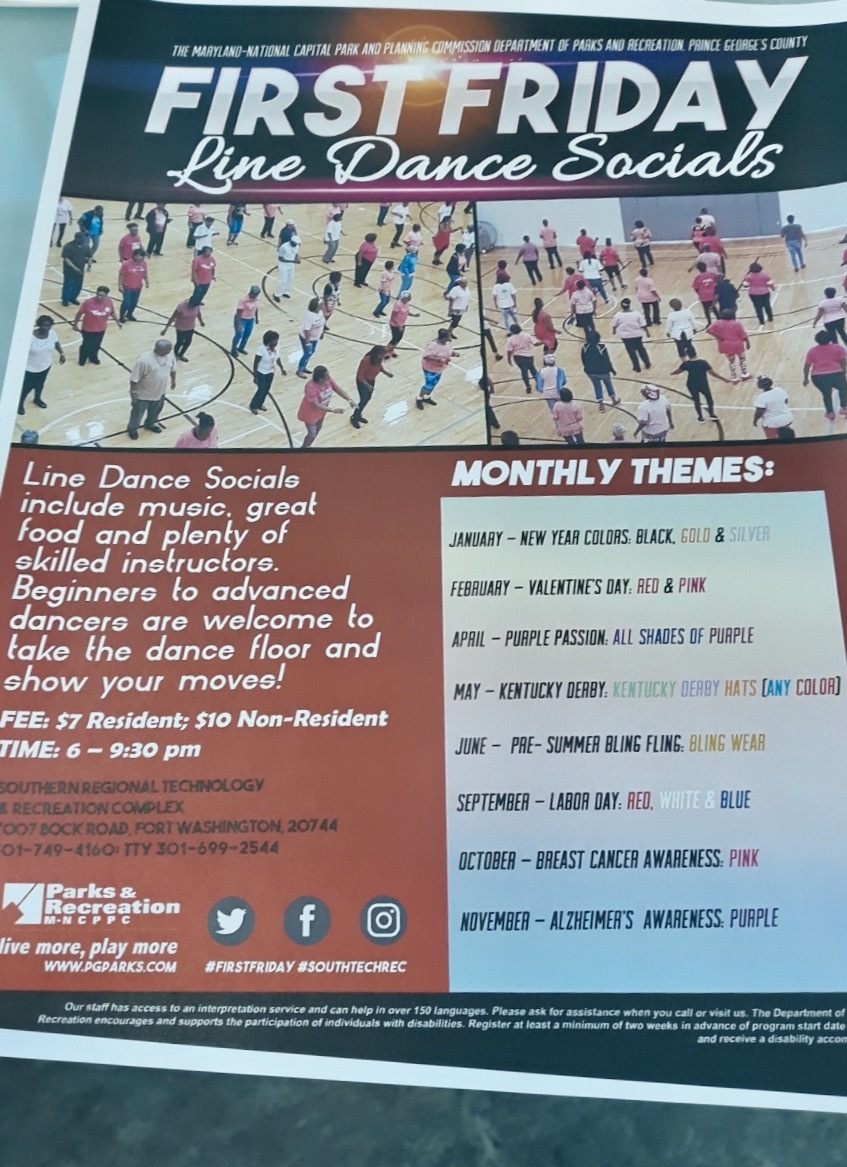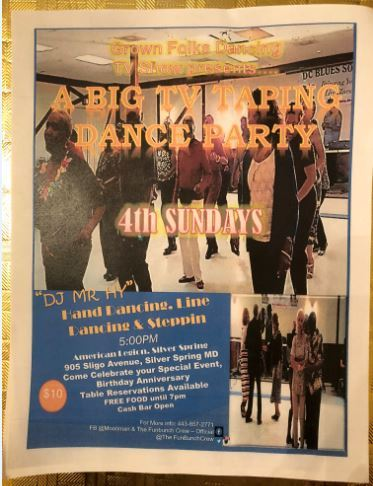table div table+table+table+table+table+table+table+table+table+table+table+table+table+table+table+table+table+table+table+table+table+table+table+table+table+table+table+table+table+table+table+table+table+table+table+table+table+table+table+table+table+table+table+table+table+table+table+table+table+table+table+table+table+table+table+table+table+table+table+table+table+table+table+table+table+table+table+table+table+table+table+table+table+table div table{width:100%;padding:0}table div table+table+table+table+table+table+table+table+table+table+table+table+table+table+table+table+table+table+table+table+table+table+table+table+table+table+table+table+table+table+table+table+table+table+table+table+table+table+table+table+table+table+table+table+table+table+table+table+table+table+table+table+table+table+table+table+table+table+table+table+table+table+table+table+table+table+table+table+table+table+table+table+table+table div table img{width:96.23%;padding:0;float:none}table div table+table+table+table+table+table+table+table+table+table+table+table+table+table+table+table+table+table+table+table+table+table+table+table+table+table+table+table+table+table+table+table+table+table+table+table+table+table+table+table+table+table+table+table+table+table+table+table+table+table+table+table+table+table+table+table+table+table+table+table+table+table+table+table+table+table+table+table+table+table+table+table+table+table div table td{width:100%;padding:0 1.88% 18px}/* styles */